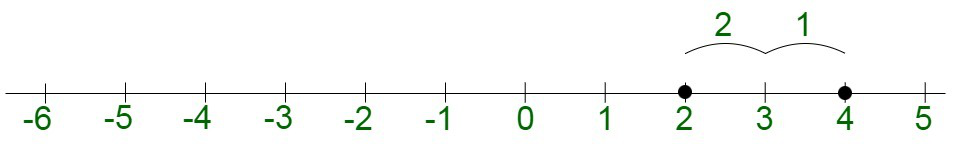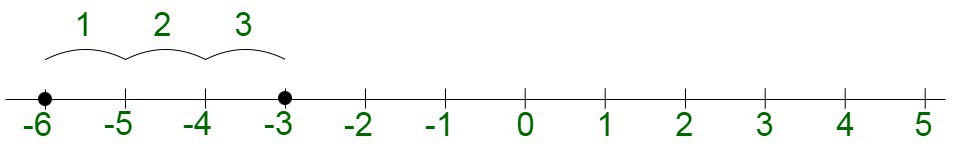GeeksforGeeks App
Open AppBrowser
Continue

# How to add positive and negative numbers?

Algebra is a special branch of mathematics that is used to deal with the arithmetic operations, such as addition, subtraction, multiplication, or division, and the associated symbols, also known as variables. The variables are not fixed and change their value. Some of the examples of variables are x,y, and z.

Zero

Zero holds a unique distinction of holding neither positive nor negative value. Zero segregates the positive numbers from the negative ones. The negative numbers lie on the left of the number line, indicated as,  –4, –3, –2, –1, 0, whereas positive numbers are, 1, 2, 3, 4.

Positive Numbers

Positive Numbers are the integral numbers that are greater than equal to zero. Positive numbers are infinite in number. In other words, any number above zero is counted as a positive number. Positive numbers lie on the right of the number line. For instance, savings are positive. Positive numbers can include rational numbers, irrational numbers, or complex numbers. The positive numbers increase in magnitude as they move farther from the number line.

Negative Numbers

Negative Numbers are the integral numbers that are less than zero. In other words, any number below zero is counted as a negative number. Negative numbers lie on the left of the number line. For instance, loans are negative. The negative numbers can include rational numbers, irrational numbers, or complex numbers. The negative numbers decrease in magnitude as they move farther from the number line.

There are three main cases that may arise due to the addition of positive and negative numbers :

The positive numbers can be easily added by performing simple addition. In the case of the addition of positive numbers, the magnitude of the numbers is simply added and is equivalent to the summation of both of their magnitudes. The resultant sign is also positive in nature.

For instance, 3 and 5 are two positive numbers, their addition is performed as :

2 + 2 = 4The negative numbers can be easily added by performing simple addition. In the case of the addition of negative numbers, the magnitude of the numbers is simply added and is equivalent to the summation of both of their magnitudes. The resultant sign is however negative in nature.

For instance, -3 and -3 are two negative numbers, there addition is performed as:

(-3) + (-3) = -6Case III: Adding positive and negative numbers

The positive and negative numbers can be added by taking their signs into consideration. The addition can then be performed by taking into consideration their magnitude. In case the positive number is greater than the negative, the overall sign of the result is positive. For example,

+5 + (-3)  = 5 – 3

= +2

The overall result is positive in nature.

Contradictorily, in case the negative number is greater, the overall sign of the result is negative. For example,

-5 + 3 = 3 – 5

= – 2

The overall result is negative in nature.

### Sample Questions

Question 1. Evaluate –  Add these negative numbers; -4 + (-8) + (-20) + (-2) + (-30)

Solution:

-4 + (-8) + (-20) + (-2) + (-30)

Open the brackets

= -4 – 8 – 20 – 2 – 30

= -64

Question 2. Evaluate – Subtract these negative numbers; -40 – (-8) – (-30) – (-18) – 2

Solution:

-40 – (-8) – (-30) – (-18) – 2

First open the brackets

= -40 + 8 + 30 + 18 – 2

= -40 – 2 + 8 + 30 + 18

= -42 + 56

= 14

Question 3. Evaluate 50 + (-4) – 26 – (-52) + 100

Solution:

50 + (-4) – 26 – (-52) + 100

First open the brackets

= 50 – 4 – 26 + 52 + 100

= 50 + 52 + 100 – 4 – 26

= 202 – 30

= 172

Question 4. Evaluate (-50 + 16 – 32) × (26 – 30 – 28)

Solution:

(-50 + 16 – 32)×(26 – 30 – 28)

First solve the brackets

= (-50 – 32 + 16)×(26 – 30 – 28)

= (66)×(-32)

= -2112

My Personal Notes arrow_drop_up
Related Tutorials